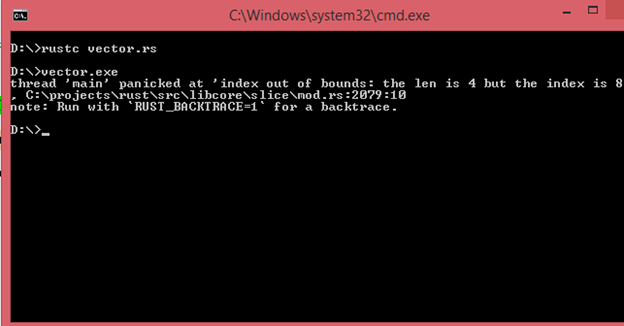# Rust向量

• 向量用於存儲相同類型的值。
• 矢量由`Vec <T>`表示。
• `Vec <T>`由標準庫提供，它可以保存任何類型的數據，其中`T`確定向量的類型。
• 向量的數據在堆上分配。
• 向量是一個可增長的數組意味着可以在運行時添加新元素。

`Vec <T>` ：當向量保持特定類型時，它在角括號中表示。

## 如何創建向量？

``Let v : Vec<i32> = Vec::new();``

Rust提供`vec!` 用於創建向量並保存提供的值的宏。

``let v = vec![10,20,30,40,50];``

``let v = vec![2 ; i];``

## 訪問元素

``````fn main()
{
let v =vec![20,30,40,50];
println!("first element of a vector is :{}",v);
println!("Second element of a vector is :{}",v);
println!("Third element of a vector is :{}",v);
println!("Fourth element of a vector is :{}",v);
}``````

``````first element of a vector is :20
Second element of a vector is :30
Third element of a vector is :40
Fourth element of a vector is :50``````

``````fn value(n:Option<&i32>)
{
match n
{
Some(n)=>println!("Fourth element of a vector is {}",n),
None=>println!("None"),
}
}
fn main()
{
let v =vec![20,30,40,50];
let a: Option<&i32>=v.get(3);
value(a);
}``````

``Fourth element of a vector is 50``

## []和get()方法的區別：

• get(index)函數
``````fn value(n:Option<&i32>)
{
match n
{
Some(n)=>println!("Fourth element of a vector is {}",n),
None=>println!("None"),
}
}
fn main()
{
let v =vec![20,30,40,50];
let a: Option<&i32>=v.get(7);
value(a);
}``````

``None``
• []運算符
``````fn main()
{
let v =vec![20,30,40,50];
println!("{}",v);
}``````## 迭代向量中的值

``````fn main()
{
let v =vec![20,30,40,50];
print!("Elements of vector are :");
for i in v
{
print!("{} ",i);
}
}``````

``Elements of vector are :20 30 40 50``

``````fn main()
{
let mut v =vec![20,30,40,50];
print!("Elements of vector are :");
for i in &mut v
{
*i+=20;
print!("{} ",i);
}
}``````

``Elements of vector are :20 30 40 50``

## 更新矢量

``````fn main()
{
let mut v=Vec::new();
v.push('j');
v.push('a');
v.push('v');
v.push('a');
for i in v
{
print!("{}",i);
}
}``````

``java``

## 刪除矢量

``````fn main()
{
let v = !vec[30,40,50];
} # => v 在這裏被釋放，因爲它超出了範圍。``````

## 使用Enum存儲多種類型

``````#[derive(Debug)]
enum Values {
A(i32),
B(f64),
C(String),
}

fn main()
{
let v = vec![Values::A(5),
Values::B(10.7),Values::C(String::from("Yiibai"))];
for i in v
{
println!("{:?}",i);
}
}``````

``````A(5)
B(10.7)
C(Yiibai)``````

• Rust在編譯時知道向量元素的類型，以確定每個元素需要多少內存。
• 當向量由一個或多個類型的元素組成時，對元素執行的操作將導致錯誤，但使用帶有匹配的枚舉將確保可以在運行時處理每個可能的情況。